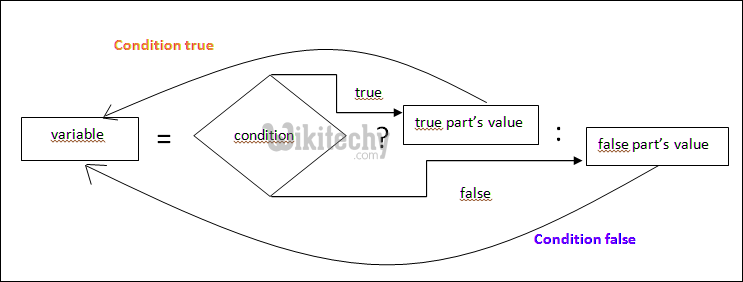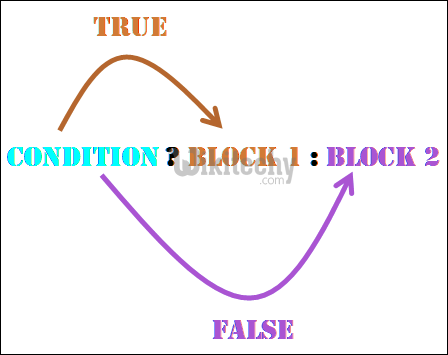# python tutorial - Ternary Operator | Ternary Operator in Python - learn python - python programmingLearn Python - Python tutorial - python ternary operator - Python examples - Python programs

Ternary operators also known as conditional expressions are operators that evaluate something based on a condition being true or false. It was added to Python in version 2.5.It simply allows to test a condition in a single line replacing the multiline if-else making the code compact.

Syntax :

1. Simple Method to use ternary operator:

python - Sample - python code :

python tutorial - Output :

1. Direct Method by using tuples, Dictionary and lambda

python - Sample - python code :

python tutorial - Output :

1. Ternary operator can be written as nested if-else:

python - Sample - python code :

Above approach can be written as:

python - Sample - python code :

python tutorial - Output :Important Points:

• First the given condition is evaluated (a < b), then either a or b is returned based on the Boolean value returned by the condition
• Order of the arguments in the operator is different from other languages like C/C++ (See C/C++ ternary operators).
• Conditional expressions have the lowest priority amongst all Python operations.Method used prior to 2.5 when ternary operator was not present
In an expression like the one given below , the interpreter checks for the expression if this is true then on_true is evaluated, else the on_false is evaluated.

Syntax :

Example :

python tutorial - Output :

Note : The only drawback of this method is that on_true must not be zero or False. If this happens on_false will be evaluated always. The reason for that is if expression is true, the interpreter will check for the on_true, if that will be zero or false, that will force the interpreter to check for on_false to give the final result of whole expression.

Wikitechy tutorial site provides you all the learn python , python download , python dictionary , python snake , python list , burmese python , download python , python book , python gui , python for , python threading